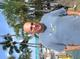• # Python 3.3 versus 3.5 perhaps? Odd behavior

I would like to give some feedback about ...

HTTP_USER_AGENT:

```Mozilla/5.0 (Macintosh; Intel Mac OS X 10.10; rv:44.0) Gecko/20100101 Firefox/44.0
```

Hello!

Anaconda doesn't offer Python 3.3, so I tried this with Python 3.5, and I get FAIL even though the tests pass locally.

Any thoughts? Here is my code: ``` def checkio(data):

```data.sort()

# if the array length is odd, return the middle number
if len(data) % 2 == 1:
return data[round(len(data) / 2)]
else:
# The array length is even. Average the middle two numbers.
middle_first = data[round(len(data) / 2) - 1]
middle_second = data[round(len(data) / 2)]
return (middle_first + middle_second) / 2
```

# These "asserts" using only for self-checking and not necessary for auto-testing

if name == 'main': assert checkio([1, 2, 3, 4, 5]) == 3, "Sorted list" assert checkio([3, 1, 2, 5, 3]) == 3, "Not sorted list" assert checkio([1, 300, 2, 200, 1]) == 2, "It's not an average" assert checkio([3, 6, 20, 99, 10, 15]) == 12.5, "Even length" print("Start the long test") assert checkio(list(range(1000000))) == 499999.5, "Long." print("The local tests are done.") ```4